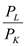# Quiz 9: Linear Programming

Business

The following explains the reason for true or false of each statement. (A) Constant returns to scale and constant input prices are the only requirements for the total cost function to be linear. This is true because a constant return to scale and input prices provides linearity to the cost function. (B) The total cost (C) function of inputs L and K can be defined as follows:The slope of the cost function is always-. If both the input prices increase, the slope won't change due to change in price. The statement that the slope always changes due to change in input prices is false. (C) The slack variables hold a property of non-negativity. Tis means the slack variables cannot be in negative. And its negative vale means, the amount of input resource employed is greater than the inputs available. The answer is true. (D) The answer is true. A production process ray explains the relationship between inputs and output. As linear programming involves only constant return to scale phase in a production process, the increase in input gives a proportionate change in output. Hence, equal distances along a production process ray in a linear programming always represent identical outputs, whereas the outputs are not identical when the production process rays are different. (E)The answer is false. The nonbinding constraints don't intersect the optimal solution. The optimal solution always lies in the feasible area. And feasible area always lies between the constrained areas in the graph.

Linear programming is used as a tool of managerial decision in many fields of business such as accounting, finance etc. Linear programming can be used to calculate and minimize cost of the firms; it can also be used to allocate resources efficiently so that the profit maximizes at minimum cost. Resource allocation, price calculation, cost minimization, and profit maximization are few examples where linear programming is used intensively.

The labor (L) and capital (K) functions of Cherry Devices Inc. are as follows:(A) The output that can be produced with 4 units of labor and 400 units of capital is:1 unit of output can be produced with 4 units of labor and 400 units of capital. Similarly, with 16units of labor and 1600 units of capital the output would be:4 units of output can be produced with 16 units of labor and 1600 units of capital. The returns to scale can be calculated by substituting the values of labor and capital in the marginal product functions of labor and capital: With 4 units of labor and 400 units of capital, the marginal product of labor:The marginal product of capital is:Both the marginal products are less than one. This means for 1% change in input, there is less than 1% change in output. Hence there are diminishing returns to scale. (B) The change in marginal product of labor as units of labor change from 4 to 16 units and capital remains at 400 units is: With 4 units of labor and 400 units of capital, the marginal product of labor:Similarly with 16 units of labor and 400 units of capital:For marginal product of capital: With 4 units of labor and 400 units of capital, the marginal product of capital:Similarly with 4 units of labor and 1600 units of capital:There are diminishing returns to scale. (C) If the inputs are to be used in the ratio 4L:\$00K or L:100K ratio with the constraints of L=4000 and K=480,000, then the output produced would be:The marginal product of labor and capital would be:Similarly, for capital:There is no answer for this question